Next: 4.2.3 Assembling Up: 4.2 Three-Dimensional Scalar Finite Previous: 4.2.1 Linear Shape Functions   Contents

## 4.2.2 Tetrahedron Barycentric Coordinates

Let P be a point inside the tetrahedral element as shown in Fig. <4.7>. It divides the tetrahedron in four sub-tetrahedrons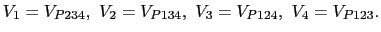The barycentric coordinates of the point P are given by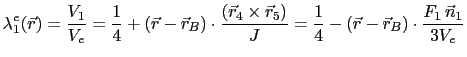(4.66)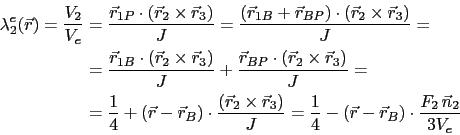(4.67)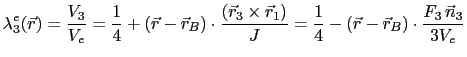(4.68)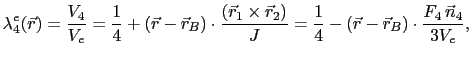(4.69)

where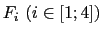is the area of the triangular face of the tetrahedron opposite to the vertex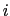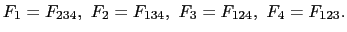The vector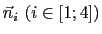is normal to its according face, has the length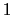and points outwards. The position vector is written as(4.70)

andis the position of the vertex.

Analogously to the two-dimensional case it can be shown that the barycentric coordinates are equal to the linear element shape functions in (4.65). Thus the same notation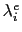is used.

The gradient of the barycentric coordinates is a constant vector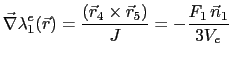(4.71)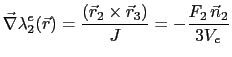(4.72)(4.73)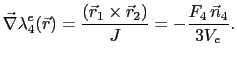(4.74)

Analogously to the two-dimensional case it is valid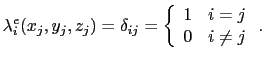(4.75)

The barycentric coordinateis constant on a plane parallel to the element facet opposite to the-th node and it is zero on this opposite facet, which ensures the inter-element continuity of the element interpolation function (4.58).

Only three of the four linear element form functions are independent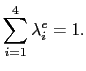(4.76)

For points inside the element and on the element facets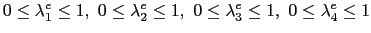(4.77)

is satisfied. Similar to the two-dimensional case some of the barycentric coordinates of a point outside the element can be negative or greater than 1.

Otherwise, the barycentric coordinates can be used to represent the coordinates of each point inside the tetrahedral element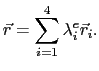(4.78)

Using (4.76), equation (4.78) leads to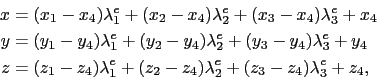(4.79)

which gives the coordinate transformation as shown in Fig. <4.8>.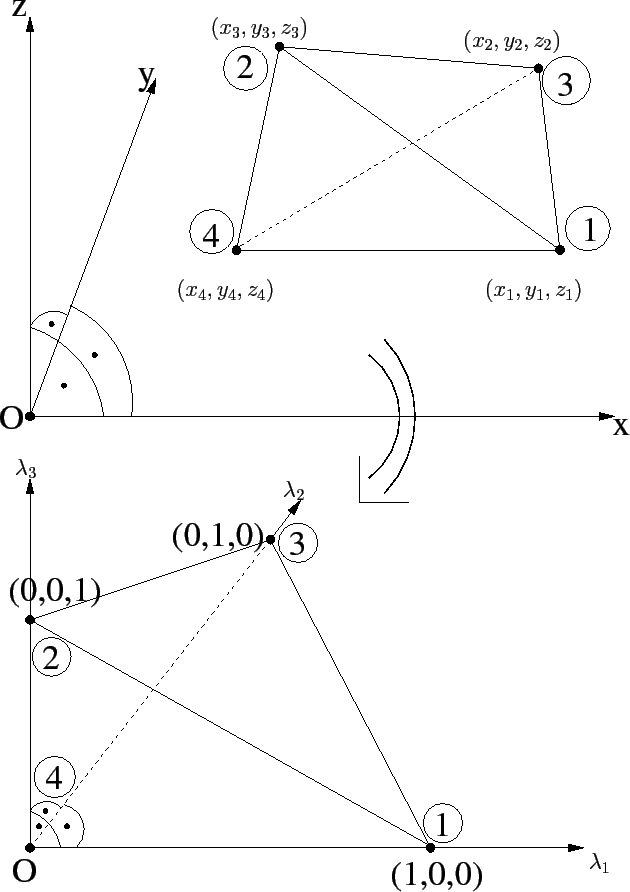Next: 4.2.3 Assembling Up: 4.2 Three-Dimensional Scalar Finite Previous: 4.2.1 Linear Shape Functions   Contents

A. Nentchev: Numerical Analysis and Simulation in Microelectronics by Vector Finite Elements